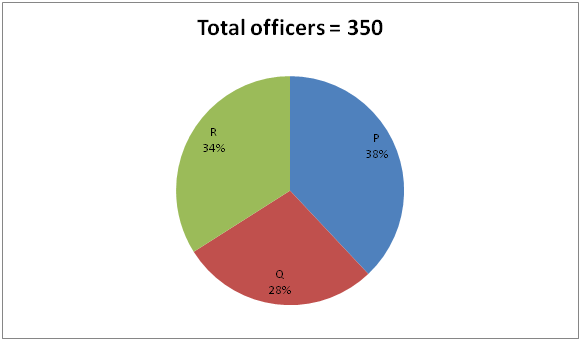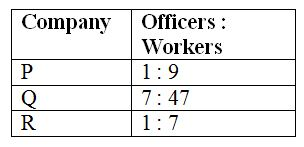# SBI PO Prelims Quantitative Aptitude Questions 2019 (Day-44)

Dear Aspirants, Our IBPS Guide team is providing new series of Quantitative Aptitude Questions for SBI PO 2019 so the aspirants can practice it on a daily basis. These questions are framed by our skilled experts after understanding your needs thoroughly. Aspirants can practice these new series questions daily to familiarize with the exact exam pattern and make your preparation effective.

[WpProQuiz 6333]

### Click Here for SBI PO Pre 2019 High-Quality Mocks Exactly on SBI Standard

1) If the heights of two cones are in the ratio of 2 : 3 and the diameters are in the ratio of 5 : 6, then find the ratio of volume of those two cones?

a) 25 : 54

b) 15 : 11

c) 33 : 25

d) 42 : 23

e) None of these

2) In a set of three numbers, the average of first two numbers is 6, the average of last two numbers is 7 and the average of first and last numbers is 8. Find the average of three numbers?

a) 10

b) 8

c) 9

d) 7

e) None of these

3) A, B, C and D enter into a partnership by investing in the ratio of 4 : 5 : 6 : 8. After 4 months, B withdraw his 40 % of the initial investment and after another 3 months, A and D increases his investment by 30 % and 50 % respectively. If at the end of the year, C gets Rs. 79200 as profit, then find the total profit of A, B, C and D?

a) Rs. 289400

b) Rs. 314600

c) Rs. 256800

d) Rs. 337200

e) None of these

4) A train crosses a platform of length 200 m in 15 sec and the same train crosses a pole in 5 sec. Find the time taken by the train to cross a tunnel of length 300 m?

a) 20 sec

b) 24 sec

c) 18 sec

d) 22 sec

e) None of these

5) 4 years ago, the age of Ramesh and 2 years hence, the age of Kathir is in the ratio of 6 : 5. The average present age of Ramesh, Kathir and Mano is 21 years. Mano is 11 years younger than Ramesh. Find the present age of Mano?

a) 21 years

b) 23 years

c) 17 years

d) 19 years

e) None of these

Directions (Q. 6 – 10): Study the following information carefully and answer the given questions.

The following pie chart shows the total number of officers in 3 different companies and the table shows the ratio of total number ofofficers to that of total number of workers in those companies also given.Note:

Total employees = Total officers + Total workers

6) Find the difference between the total number of officers in company P to that of total number of workers in company R?

a) 700

b) 950

c) 1050

d) 1200

e) None of these

7) Total number of employees in company Q is approximately what percentage of total number of employees in company R?

a) 65 %

b) 50 %

c) 110 %

d) 80 %

e) 95 %

8) Find the average number of workers in all the given companies together?

a) 752

b) 896

c) 678

d) 944

e) None of these

9) Total number of officers in company Q and R together is approximately what percentage of total number of employees in company Q?

a) 60 %

b) 70 %

c) 30 %

d) 45 %

e) 15 %

10) Find the ratio between the total number of workers in company Q to that of total number of employees in company P?

a) 329 : 665

b) 152 : 347

c) 29 : 43

d) 7 : 19

e) None of these

The ratio of heights of two cones (h1 and h2) = 2 : 3 (2x, 3x)

The ratio of diameters of two cones = 5 : 6

The ratio of radius of two cones (r1 and r2) = (5/2) : 3 [5y/2, 3y]

The volume of cone = (1/3)*πr2h

Required ratio = [(1/3)*π*r12 *h1] :[(1/3)*π*r22 *h2]

= > [(5y/2)2 * 2x] : [(3y)2 * 3x]

= >25 : 54

Let the numbers be x, y and z,

The average of first two numbers = 6

(x + y) = 12

The average of last two numbers = 7

(y + z) = 14

The average of first and last numbers = 8

(x + z) = 16

2*(x + y + z) = 12 + 14 + 16 = 42

x + y + z = 21

Required average = (21/3) = 7

The share of A, B, C and D,

= > [4*7 + 4*(130/100)*5] : [5*4 + 5*(60/100)*8] : [6*12] : [8*7 + 8*(150/100)*5]

= > [28 + 26] : [20 + 24] :  : [56 + 60]

= >27 : 22 : 36 : 58

36’s = 79200

1’s = 2200

Total profit = 143’s = Rs. 314600

The same train crosses a platform and pole. So, Speed is equal.

Speed = Distance/Time

(T.L + 200)/15 = T.L/5

T.L + 200 = 3*T.L

2*T.L = 200

T.L = 100 m

The time taken by the train to cross a tunnel

Time = Distance/Speed

Required time = (100 + 300)/(100/5) = (400*5)/100 = 20 sec

4 years ago, the age of Ramesh and 2 years hence, the age of Kathir is in the ratio of = 6 : 5 (6x, 5x)

Present age of Ramesh and Kathir  = 6x + 4, 5x – 2

The average present age of Ramesh, Kathir and Mano = 21 years

Total present age of Ramesh, Kathir and Mano = 21*3 = 63

Present age of Mano = 6x + 4 – 11 = 6x – 7

According to the question,

6x + 4 + 5x – 2 + 6x – 7 = 63

17x – 5 = 63

17x = 68

x = 4

The present age of Mano = 6x – 7 = 24 – 7 = 17 years]

Direction (6-10) :

The total number of officers in company P

= >350*(38/100) = 133

The total number of workers in company R

= >350*(34/100)*7 = 833

Required difference = 833 – 133 = 700

Total number of employees in company Q

= >350*(28/100)*(54/7) = 756

Total number of employees in company R

= >350*(34/100)*8 = 952

Required % = (756/952)*100 = 79.4 % = 80 %

The average number of workers in all the given companies together

= >[350*(38/100)*9 + 350*(28/100)*(47/7) + 350*(34/100)*7]/3

= > (1197 + 658 + 833)/3 = 896

Total number of officers in company Q and R together

= >350*(62/100) = 217

Total number of employees in company Q

= >350*(28/100)*(54/7) = 756

Required % = (217/756)*100 = 28.7 % = 30 %

The total number of workers in company Q

= >350*(28/100)*(47/7) = 658

The total number of employees in company P

= >[350*(38/100)*10] = 1330

Required ratio = 658 : 1330 = 329 : 665